# SCIENTIFIC NOTATION Scientific Notation In science we typically

• Slides: 12SCIENTIFIC NOTATIONScientific Notation • In science, we typically see very LARGE or very small numbers in our calculations. • Scientific notation is used to reduce the errors when counting ZEROES.Scientific Notation • In order to make this easier, scientists express numbers in scientific notation, which uses powers of 10.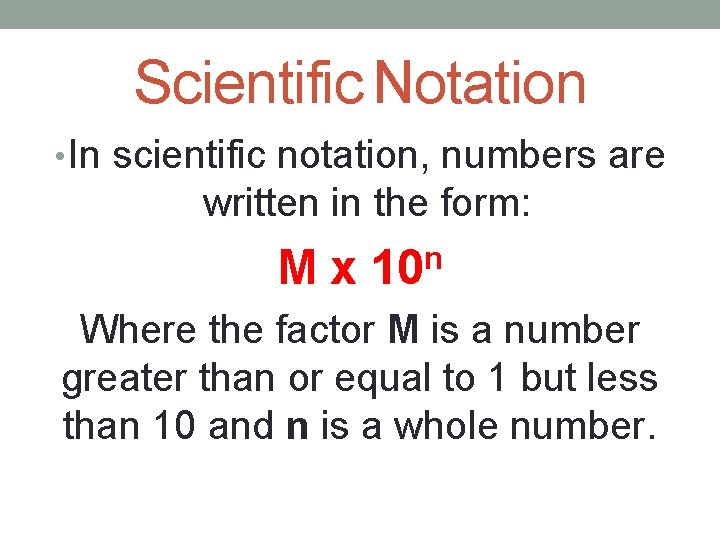Scientific Notation • In scientific notation, numbers are written in the form: M x 10 n Where the factor M is a number greater than or equal to 1 but less than 10 and n is a whole number.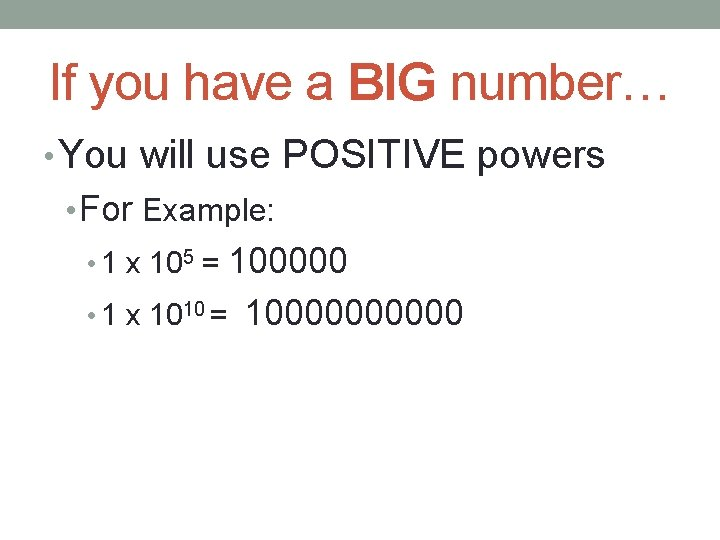If you have a BIG number… • You will use POSITIVE powers • For Example: 100000 • 1 x 1010 = 100000 • 1 x 105 =If you have SMALL numbers… • You will use NEGATIVE powers • For example: • 1 x 10 -5 = 0. 00001 • 1 x 10 -10 = 0. 000001Rules 1. Move the decimals over so that only one nonzero is to the left of the decimal. 5, 500, 000 5. 50000 0. 000067 00006. 7Rules 2. Count how many spaces you moved the decimal. • If you move to the left it’s positive. If you move to the right it’s negative. 5. 50000 moved 9 spaces left (+9) 00006. 7 moved 9 spaces right (-9)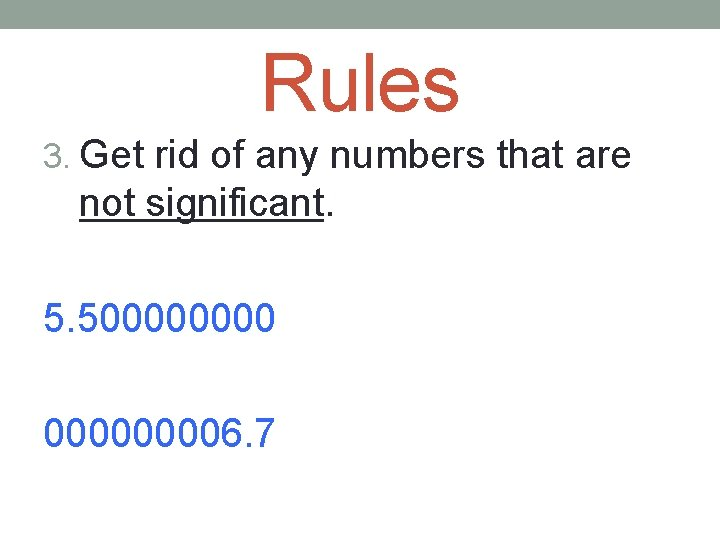Rules 3. Get rid of any numbers that are not significant. 5. 50000 5. 5 00006. 7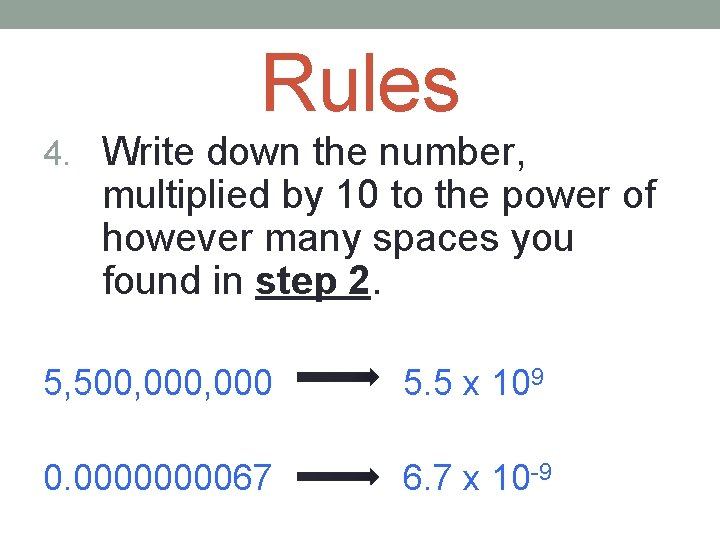Rules 4. Write down the number, multiplied by 10 to the power of however many spaces you found in step 2. 5, 500, 000 5. 5 x 109 0. 000067 6. 7 x 10 -9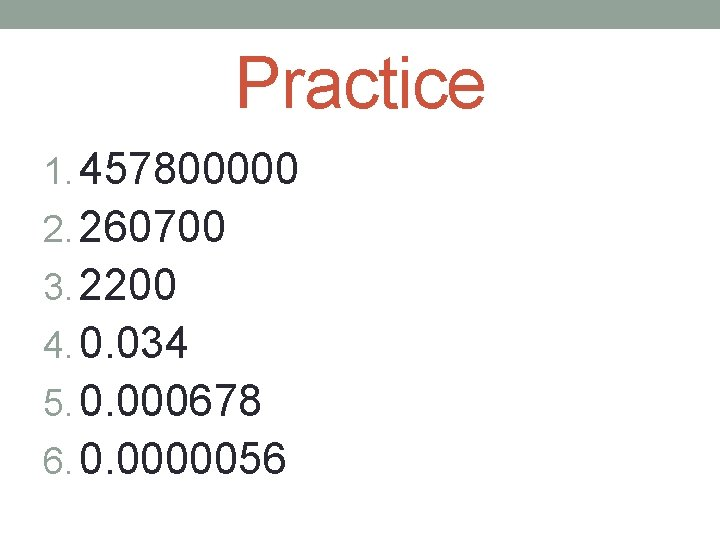Practice 1. 457800000 2. 260700 3. 2200 4. 0. 034 5. 0. 000678 6. 0. 0000056 4. 578 x 108 2. 607 x 105 3 2. 2 x 10 3. 4 x 10 -2 -4 6. 78 x 10 5. 6 x 10 -6Scientific Notation -> Standard Form 1. 5. 89 x 10 -2 2. 4. 932 x 10 -1 3. 9. 7 x 10 -6 4. 6. 01 x 107 5. 2. 485 x 105 6. 1. 45 x 103 0. 0589 0. 4932 0. 0000097 60100000 248500 1450